# Section 6.1. Conditional Pixel Changes

[Page 173 (continued)]

### 6.1. Conditional Pixel Changes

So far we have been processing all of the pixels in the same way. But what if we want to process the pixels in different ways? For example, we might want to turn someone's hair a different color, or get rid of "red-eye" in a picture, or even reduce the number of colors in a picture.

[Page 174]

We need something that executes a block of code only if some condition (expression) is true. We know that computers can compare values to see if they are equal, less than, or greater than (and combinations of these) and return true or false. We have used this with loops by continuing a loop while the index is less than the length of the array as in: while(index < pixelArray.length). This will execute the loop while the expression (index < pixelArray.length) is true.

Try the following in the interactions pane:

`> 0 < 20 true > 30 < 20 false > 20 < 20 false > 20 <= 20 true`

One way to conditionally execute code in Java is with an if (expression). The if is a keyword that means that if the expression in the parentheses evaluates to true then execute the following statement or block of statements. If it is false just skip the execution of that statement or block of statements (Figure 6.1) So, we can check whether the color at the current pixel is close to a particular color and if so execute a statement or block of statements (in curly braces).

##### Figure 6.1. Flowchart of an if statement.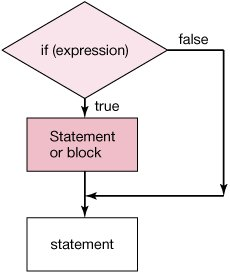Here is an example if statement with just a single statement indented on the next line following the if. We usually indent statements following an if to show that they are part of the statement and will not always be executed. Java doesn't care about the indentation, but it makes it easier for people to read and understand.

`[Page 175]boolean flag = true; if (flag)    System.out.println("flag is true"); System.out.println(flag);`

Let's try the following in the interactions pane:

`> int x = 30; > if (x < 40) System.out.println("x is less than 40"); x is less than 40 > if (x > 40) System.out.println("x is greater than 40"); > System.out.println(x); 30`

Notice that since x is less than 40, the string saying so was output. However, since x is not greater than 40, the string saying x is greater than 40 was not output. We do see the output from the next statement System.out.println(x) since execution jumps to the statement following an if when the expression is false.

#### 6.1.1. Comparing Colors

What does it mean to compare two colors? How can the computer tell if the color at the current pixel is "red"? The distance between two colors is the Cartesian distance between the colors as points in a three-dimensional space, where red, green, and blue are the three dimensions. Recall that the distance between two points (x1, y1) and (x2, y2) is:The similar measure for two colors (red1, green1, blue1) and (red2, green2, blue2) is: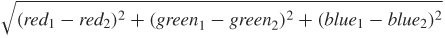However, you won't have to code this. The Pixel class has an object method colorDistance(Color color) which returns the distance between the color in the current Pixel object and the passed color. The hard part is determining what "close enough" is for two colors.

#### 6.1.2. Replacing Colors

Here's a program that tries to replace the brown color with red. Mark used the picture explorer to figure out roughly what the RGB values were for Katie's brown hair, then wrote a program to look for colors close to that, and then increased the redness of those pixels. Mark played a lot with the value that he used for distance (here, 50.0) and the amount of redness increased (here, 100% increase). However, this approach turned part of the couch and carpet red too (Figure 6.2).

[Page 176]

##### Figure 6.2. Increasing reds in the browns.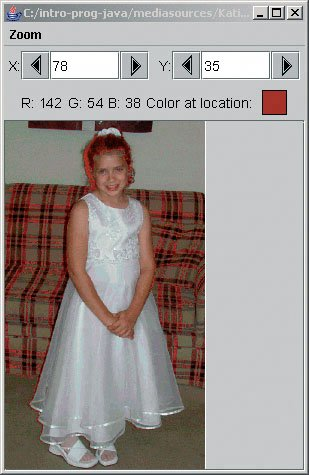##### Program 33. Color Replacement: Turn Brown into Red`/** * Method to turn the brown in a picture * into red */ public void turnBrownIntoRed() { Color brown = new Color(42,25,15); Pixel[] pixels = this.getPixels(); Pixel pixel = null; // loop through the pixels for (int i=0; i

[Page 177]

To use this method to turn Katie into a redhead, first create a Picture object from the file "KatieFancy.jpg". Then invoke the method turnBrownIntoRed on that Picture object and show or explore the result.

`> String fileName = FileChooser.getMediaPath("KatieFancy.jpg"); > Picture picture = new Picture(fileName); > picture.turnBrownIntoRed(); > picture.explore();`

Notice that we can use a simple for loop through the one-dimensional array of pixels for this. We don't care where the pixels are in the two-dimensional array in this method. Of course, we could have used nested for loops instead to loop through all the pixels.

What this method is doing is looping through all the pixels in the current picture and for each pixel checking if the distance between the color in the current pixel is less than 50 away from the color brown (defined as red=42, green=25, blue=15). If the distance between the current color and the defined brown is less than 50, the red value at the current pixel is doubled. If the distance is equal to or greater than 50, the pixel color is not changed.

With the picture explorer we can also figure out the coordinates just around Katie's face, and then just do the browns near her face. The effect isn't too good, though it's clear that it worked. The line of redness is too sharp and rectangular (Figure 6.3).

##### Figure 6.3. On left the couch color changes, on right the couch color doesn't change. (This item is displayed on page 178 in the print version)##### Program 34. Color Replacement in a Rectangular Area`/** * Method to turn brown to red inside of * a rectangular area */ public void turnBrownToRedInRectangle() { Color brown = new Color(42,25,15); Pixel pixel = null; // loop through the x values for (int x=63; x < 125; x++) { for (int y=6; y < 76; y++) { // get the current pixel pixel = this.getPixel(x,y); // check if in dist to brown and if so double the red if (pixel.colorDistance(brown) < 50.0) pixel.setColor(new Color((int) (pixel.getRed() * 2.0), pixel.getGreen(), pixel.getBlue())); } } }`

[Page 178]

To use this method to turn Katie's hair red, first create a Picture object from the file "KatieFancy.jpg". Then invoke the method turnBrownIntoRedInRectangle on that Picture object and show the result (see Figure 6.3).

`> String fileName = FileChooser.getMediaPath("KatieFancy.jpg"); > Picture picture = new Picture(fileName); > picture.turnBrownIntoRedInRectangle(); > picture.explore();`

We put the values for the range right inside the method

`turnBrownIntoRedInRectangle()`

this meant that we didn't need to pass any parameters to specify the range but it makes the method less reusable. If we want to use it to change a different picture, we would have to edit the method to change the range and then recompile. The method would be easier to reuse if we specified the range when we invoke the method. We can also pass in the distance to check for between brown and the current color.

[Page 179]

##### Program 35. Color Replacement with Passing in the Range`/** * Method to turn brown to red in a rectangular area * specified * by startX, endX-1, startY, endY-1 * @param startX the starting location to check in x * @param endX the last pixel checked is one less than * this in x * @param startY the starting location to check in y * @param endY the last pixel checked is one less than * this in y */ public void turnBrownToRedInRectangle(int startX, int endX, int startY, int endY, double distance) { Color brown = new Color(42,25,15); Pixel pixel = null; // loop through the x values for (int x=startX; x < endX; x++) { for (int y=startY; y < endY; y++) { // get the current pixel pixel = this.getPixel(x,y); /* check if in distance to brown is less than * the passed distance and if so double the red */ if (pixel.colorDistance(brown) < distance) pixel.setColor(new Color((int) (pixel.getRed() * 2.0), pixel.getGreen(), pixel.getBlue())); } } }`

Can you think of any other things that you could do to make this method easier to reuse? What if we want to change something other than brown? What if we want to change the old color by increasing the green?

#### 6.1.3. Reducing Red-Eye

"Red-eye" is the effect where the flash from the camera bounces off the back of the subject's eyes. Reducing red-eye is a really simple matter. We find the pixels that are "pretty close" to red (a distance from red of 167 works well), then change those pixels' color to a replacement color.

[Page 180]

We probably don't want to change the whole picture. In the Figure 6.4, we can see that Jenny is wearing a red dresswe don't want to wipe out that red, too. We'll fix that by only changing the area where Jenny's eyes are. Using the picture explorer, we find the upper-left and lower-right corners of her eyes. Those points were (109, 91) and (202, 107).

##### Figure 6.4. Finding the range of where Jenny's eyes are red.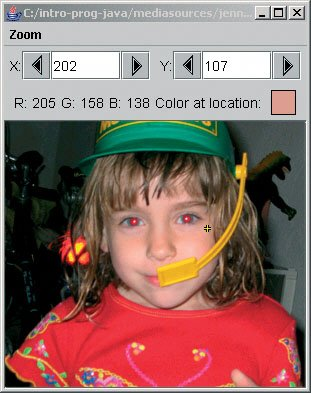##### Program 36. Remove Red-Eye (This item is displayed on pages 180 - 181 in the print version)`/** * Method to remove red-eye from the current picture object * in the rectangle defined by startX, startY, endX, endY. * The red will be replaced with the passed newColor * @param startX the top left corner x value of a rectangle * @param startY the top left corner y value of a rectangle * @param endX the bottom right corner x value of a * rectangle * @param endY the bottom right corner y value of a * rectangle * @param newColor the new color to use */ public void removeRedEye(int startX, int startY, int endX, int endY, Color newColor) { Pixel pixel = null; [Page 181] /* loop through the pixels in the rectangle defined by the startX, startY, and endX and endY */ for (int x = startX; x < endX; x++) { for (int y = startY; y < endY; y++) { // get the current pixel pixel = getPixel(x,y); // if the color is near red then change it if (pixel.colorDistance(Color.red) < 167) pixel.setColor(newColor); } } }`

We call this method with:

`> String fileName =    "c:/intro-prog-java/mediasources/jenny-red.jpg"; > Picture jennyPicture = new Picture(fileName); > jennyPicture.removeRedEye(109,91,202,107,java.awt.Color.black); > jennyPicture.explore();`

to replace the red with blackcertainly other colors could be used for the replacement color. The result was good, and we can check that the eye really does now have all-black pixels (Figure 6.5).

##### Figure 6.5. After fixing red-eye.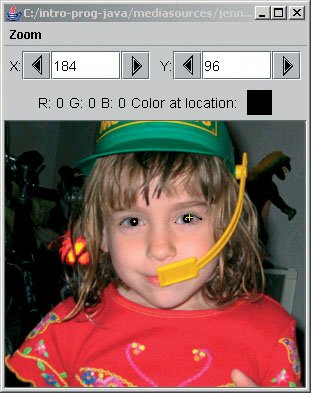Introduction to Computing & Programming Algebra in Java(c) A Multimedia Approach
ISBN: N/A
EAN: N/A
Year: 2007
Pages: 191

flylib.com © 2008-2017.
If you may any questions please contact us: flylib@qtcs.net